Accelerated MRI with CIRcular Cartesian UnderSampling (CIRCUS): a variable density Cartesian sampling strategy for compressed sensing and parallel imaging
Original Article

# Accelerated MRI with CIRcular Cartesian UnderSampling (CIRCUS): a variable density Cartesian sampling strategy for compressed sensing and parallel imaging

Jing Liu1, David Saloner1,2

1Department of Radiology and Biomedical Imaging, University of California San Francisco, San Francisco, California, USA; 2Radiology Service, VA Medical Center, San Francisco, California, USA

Corresponding to: Jing Liu. 185 Berry St, Suite 350, Radiology and Biomedical Imaging, University of California San Francisco, San Francisco, CA 94107, USA. Email: jing.liu@ucsf.edu.

Purpose: This study proposes and evaluates a novel method for generating efficient undersampling patterns for 3D Cartesian acquisition with compressed sensing (CS) and parallel imaging (PI).

Methods: Image quality achieved with schemes that accelerate data acquisition, including CS and PI, are sensitive to the design of the specific undersampling scheme used. Ideally random sampling is required to recover MR images from undersampled data with CS. In practice, pseudo-random sampling schemes are usually applied. Radial or spiral sampling either for Cartesian or non-Cartesian acquisitions has been using because of its favorable features such as interleaving flexibility. In this study, we propose to undersample data on the ky-kz plane of the 3D Cartesian acquisition by circularly selecting sampling points in a way that maintains the features of both random and radial or spiral sampling.

Results: The proposed sampling scheme is shown to outperform conventional random and radial or spiral samplings for 3D Cartesian acquisition and is found to be comparable to advanced variable-density Poisson-Disk sampling (vPDS) while retaining interleaving flexibility for dynamic imaging, based on the results with retrospective undersampling. Our preliminary results with the prospective implementation of the proposed undersampling strategy demonstrated its favorable features.

Conclusions: The proposed undersampling patterns for 3D Cartesian acquisition possess the desirable properties of randomization and radial or spiral trajectories. It provides easy implementation, flexible sampling, and high accuracy of image reconstruction with CS and PI.

Keywords: Undersampling; acceleration; random; variable-density; compressed sensing (CS); parallel imaging (PI)

Submitted Jan 02, 2014. Accepted for publication Feb 12, 2014.

doi: 10.3978/j.issn.2223-4292.2014.02.01

## Introduction

Compressed sensing (CS) and parallel imaging (PI) have been exploited to reduce scan time by undersampling k-space (1-7). This is highly desirable for 3D or 4D MR applications that usually have unreasonably long scan times. To recover images from undersampled data, incoherent random undersampling is desired for CS and certain PI methods (4,7), although in practice pseudo-random undersampling is commonly applied. Poisson-Disk sampling (PDS) provides an even but random distribution of samples (8,9), which is suited for CS&PI (7). Variable-density PDS (vPDS), which samples more densely at low frequencies, has been applied for improved fidelity of the reconstructed images (10). The computational cost for generating PDS or vPDS is relatively high, thus fast algorithms (9,11) are generally applied to remove the limit of its practical applications especially in real-time rendering. Non-Cartesian trajectories (such as radial and spiral) have been used for undersampling k-space (12-16), giving flexibility in interleaving and in controlling the undersampling factor. Similarly radial or spiral sampling patterns of the phase-encoding trajectories (on ky-kz plane), have also been explored for 3D Cartesian acquisitions (17-19).

This study proposes a novel method for generating undersampling patterns for 3D Cartesian acquisitions. It integrates the desirable features of the random and radial or spiral samplings, resulting in high accuracy of image reconstruction with CS&PI as well as sampling flexibilities. It is favorable for dynamic applications that usually involve interleaving of the sampling. In this study, we introduce the algorithm for generating a series of different sampling patterns, including radial, spiral or randomized sampling patterns, and also show its ability to accommodate interleaving for dynamic or real-time imaging. We compared our proposed sampling method with the conventional uniform random sampling (uRS), PDS, and vPDS patterns, based on retrospective undersampling on the fully sampled data sets. Images were reconstructed and compared using either CS (4) or PI (7). We showed that the proposed method is easy to implement in practice, and that it has flexible sampling patterns with high image reconstruction accuracy using CS&PI. We demonstrated images obtained with the prospectively implemented undersampling strategy.

## Methods

### Undersampling on ky-kz

The proposed method designs sampling patterns on the ky-kz plane of 3D Cartesian acquisitions (where kx is the frequency encoding axis). To undersample k-space, only a subset of all ky-kz points are acquired. To decide the locations of the points to sample and what order the sample points should follow, we introduce our CIRcular Cartesian UnderSampling (CIRCUS) method. First, we consider the case where the fully sampled matrix on the ky-kz plane has a size of N×N. This matrix can be decomposed into N/2 nested squares where the central square, with size 2×2, contains the lowest spatial frequencies, and the outermost square, of size N×N, contains the highest spatial frequencies. CIRCUS selects sampling points along the perimeter of each square based on either a uniform or golden-ratio profile (20). By shifting the sampling points along the perimeter of the square with specific offsets, it can generate different trajectories such as radial or spiral lines, or even randomized patterns.

For each of the N/2 squares:

J: size of the square, J=2,3,,N, i.e., the number of points along one side of the square;

K: total number of points along the perimeter of the square K=4·J-4;

M: number of sampling points placed on the perimeter of each square. M could be a constant for all squares or vary according to the size of each square M(J);

r: golden ratio

### CIRCUS: base

The points around the perimeter of each square are assigned indices from 0 to K-1. Since the sampling pattern advances circularly along the perimeter, the first point (with index 0) can be chosen at any point on the perimeter but needs to be consistent for all the squares, such as the point at the bottom-left corner of the square, the middle point on the left side of the square, and so on. The points can be in either clockwise or anticlockwise order. In this study, the points were chosen to be indexed in clockwise order. The golden-ratio profile has been proposed for achieving sampling flexibility (20) and broadly used in many applications especially for dynamic or real-time imaging (20-22). In this study, the indices of the selected sampling points along the perimeter of the square, based on the golden-ratio profile, are calculated as:

i=floor[mod(mr–1,1)•K] 

where the floor function provides the round-down integer value, the mod function is the modulo operator, and m=0, 1,, M–1. With Eq. , the indices of the M selected points are within 0 to K-1 and distributed nearly uniformly around the perimeter of the square due to the property of the golden-ratio profile.

Examples are shown in Figure 1, where the sampling matrix size is N=32 and the number of selected points is M=16. In Figure 1A, four representative squares of size J=6, 16, 24 and 32 (from center to periphery) are shown. It can be noted that the M=16 selected points are approximately evenly distributed around the perimeter of each square. The entire k-space sampling pattern corresponding to that shown in Figure 1A are displayed in Figure 1B, with an acceleration factor of R=4.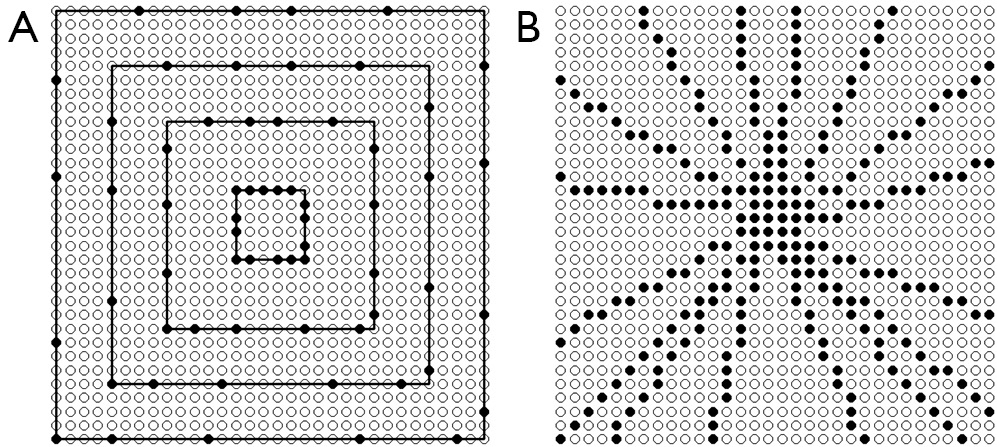Figure 1 Circular undersampling on ky-kz plane (the sampling matrix N×N =32×32; the number of selected points M=16). (A) Four representative squares are highlighted with solid lines. CIRCUS selects the points along the perimeter of each square starting at the bottom-left corner, in clock-wise order and based on the golden-ratio profile, resulting in an approximately uniform distribution along the perimeters of the representative squares; (B) The corresponding entire ky-kz CIRCUS base pattern with an undersampling factor of 4.

A constant value M for all the squares results in a variable-density sampling pattern: denser at the k-space center and less dense at the high frequencies. This is similar to a radial sampling pattern, where the value of M corresponds to the number of radial lines (spokes). M can be made to vary according to the square size J, e.g., M = a·J, where a is a constant, resulting in an undersampling pattern that is more uniform across the low and high frequencies. In this study, we only investigated the variable-density CIRCUS patterns. Overall, M, the number of the selected points for each square, controls the density and degree of undersampling. The sampling pattern described above was attained with the golden-ratio profile. Similarly, a uniform radial pattern is generated if CIRCUS uniformly advances around the perimeter of each square. In this study, the golden-ratio profile was applied for all CIRCUS patterns.

### CIRCUS: randomization with shifting

The above described radial-like under sample pattern (CIRCUS base) contains lines which are nearly straight lines in the k-space. To spread out the points in the sampling pattern, we break the radial lines into smaller pieces by applying specific shifting to the sampling points along the radial lines:

i'=floor{mod[(m+s1)•r–1,1]•K} 

where s1=b·J denotes the shift and b is an integer to adjust the degree of randomization of the sampling points (b=0, 1, 2,).

The points along a radial line with CIRCUS base are spread out in k-space after shifting is applied (Figure 2A), where N=128, m=0, and b=0, 3, 12 and 30 respectively. Figure 2B shows four representative ky-kz sampling patterns with M=32, achieving the same acceleration factor of R=8. Figure 2C shows the corresponding point-spread functions (PSF) (window level rescaled with power of 0.5). Randomized radial patterns tend to have reduced energy in the sidelobes of the PSF (Figure 2C). Based on the sampling patterns generated with CIRCUS, it was empirically found that b needed to be larger enough (≥30) to provide sufficient randomization to the sampling pattern. In the Results Section, we will investigate sampling patterns with a series of values of b and find out the reliable values for achieving image reconstruction with high accuracy. Since this type of sampling patterns follow radial or broken down radial patterns, we call them CIRCUS radial patterns.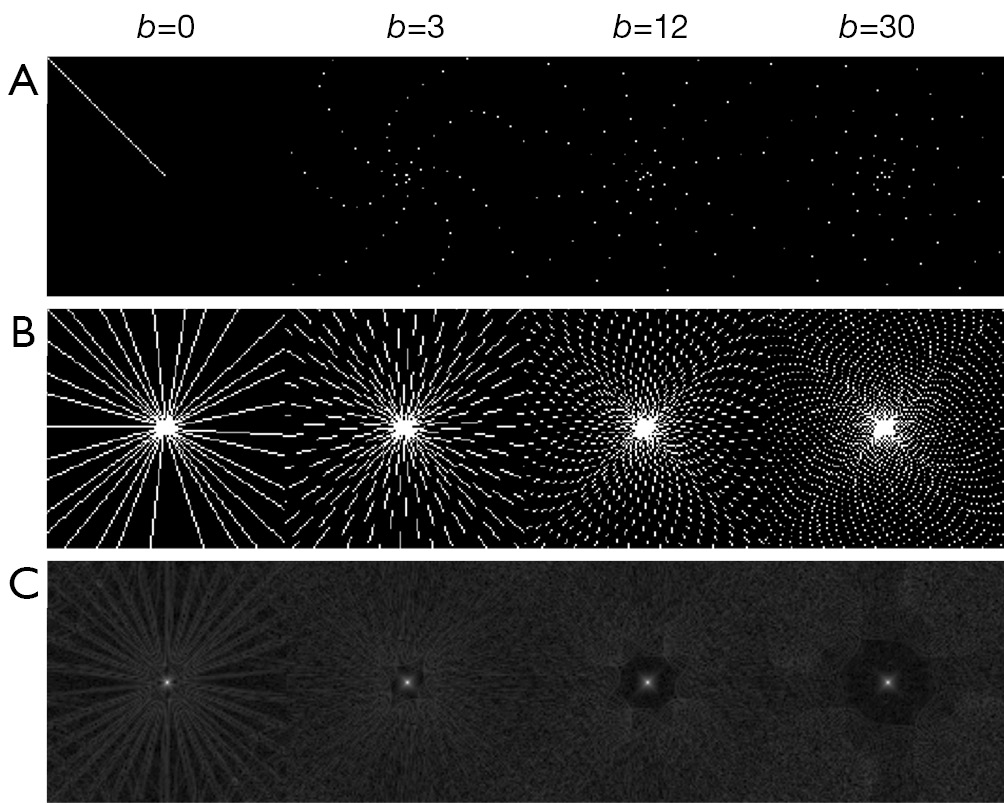Figure 2 CIRCUS radial. (A) A representative radial line and the corresponding patterns with shifting {Eq. , b=0, 3, 12 and 30 respectively}; (B) four representative CIRCUS radial patterns with the same undersampling factor of R=8; (C) the corresponding point spread functions contain less energy in the sidelobes as the degree of sampling randomization increases.

#### Type 2-CIRCUS spiral

By applying shifting, we can break down the radial lines thus introduce randomization to the sampling distribution as described above. Another way for introducing randomization is to turn the radial lines into spirals. For the indices obtained above with Eq. , at each square a shift (denoted as s2) that is nonlinearly varying according to J, the size of each square, is introduced as:

i''=mod(i+s2,K) 

where s2=ceil(Jc)–1, ceil is the function that returns the rounded up value of the argument and c is a constant to control the shifting (1<c<2). The nonlinear shifts will make the radial lines generated with Eq.  twist to form a spiral shape.

The radial line obtained with CIRCUS base are turned into spirals after shifting with Eq. , showed in Figure 3A, where N=128, m=0, c=1.3, 1.5, 1.7, and 1.9 respectively. As shown, the parameter c determines the degree of twisting and thus controls the degree of randomization. Four representative ky-kz sampling patterns with M=32 (R=8) and their corresponding PSFs are displayed in Figure 3B,C. The individual spiral leaf becomes more randomized, as c gets closer to 2. However, the degree of randomization across the entire sampling pattern also depends on the number of points M (or say, the acceleration factor). A choice of c=1.5 was empirically found to consistently provide a reasonable randomization of the sampling points and low energy at the PSF sidelobes for different acceleration factors.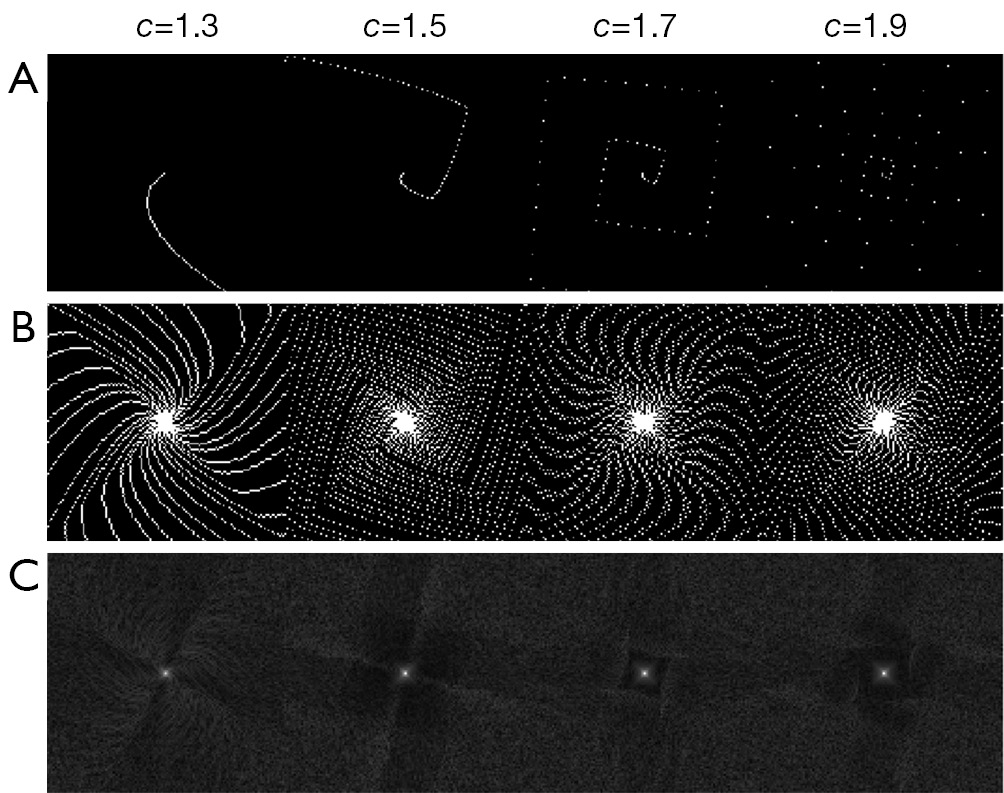Figure 3 CIRCUS spiral. (A) Representative spiral leaves with shifting {Eq. , c=1.3, 1.5, 1.7 and 1.9 respectively}; (B) four representative CIRCUS radial patterns with R=8; (C) the corresponding point spread functions contain less energy in the sidelobes as the degree of sampling randomization increases.

### Sampling order & interleaving

In addition to permitting flexible undersampling patterns as demonstrated above, CIRCUS also has flexibility for choosing a favourable sampling order. It is possible to acquire all the selected sample points along the perimeter of one square before switching to another square. The order of sampling of the squares could be from the edge to center, center to edge, or in any arbitrary order.

The sampling order could also be chosen similarly to what is done for radial or spiral patterns, i.e., acquire a radial line or spiral leaf that acquires one point from each square through all the squares before switching to another line or leaf. This sampling order is very useful for interleaving k-space for time-resolved or real-time imaging applications such as is done in true radial or spiral sampling. CIRCUS can also benefit from acquiring 3D k-space centers throughout the entire scan, resulting in potential motion robustness or motion tracking as demonstrated previously in many applications with k-space centers acquired throughout the scan (12,15,23,24).

A time-resolved interleaving scheme is implemented as a modification to Eq. :

i'''=floor{mod[(m+t•M)•r–1,1]•K} 

where t is the time index (t=0, 1,…), and M, the number of the selected points also denotes the number of sampling points per time frame (interleave). M could be flexibly chosen for specific applications. When M is chosen as 1, Eq.  is simplified to generate a continuous sampling pattern where a line or leaf of a unique angle is acquired at each time point. This is well suitable for dynamic or real-time imaging, since the unique characteristics of the golden-ratio sampling profile provide approximately uniform k-space sampling at any time frame and allow for robust sliding window reconstructions with variable temporal resolution and arbitrary duration (20).

For N=128, M=32, the sampling patterns of four sequential time-points (t=0, 1, 2, 3) with the acceleration factor of R=8 are displayed in Figure 4A. The patterns that result for randomized schemes with Eq.  (b=30) and Eq.  (c=1.5) are also shown in Figure 4B,C. The last columns are the combinations of all the sampling points from the corresponding four time-points. Due to the unique property of the golden-ratio profile, the sampling points are well interleaved through different time frames. The randomized schemes (Figure 4B,C) provide a more spread out distribution of the sampling points compared to that shown in Figure 4A.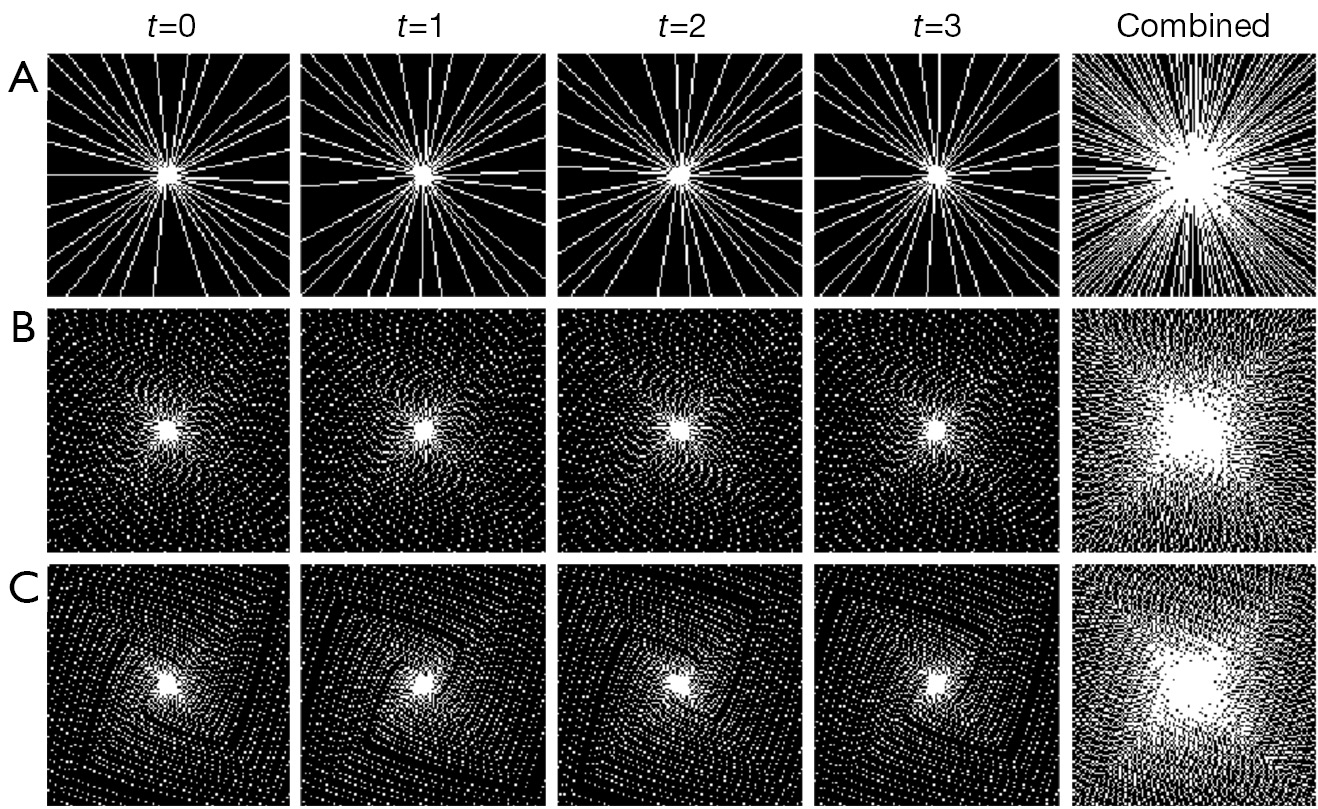Figure 4 Representative sampling patterns at four sequential time frames (shown in the first four columns) generated with: (A) Eq. ; and randomization obtained with (B) Eqs. [2,4 (CIRCUS radial, b=30); and (C) Eqs. [3,4 (CIRCUS spiral, c=1.5). For each of the three cases, the combinations of the four time frames are shown in the last column.

### Arbitrary ky-kz sampling matrix size & partial acquisition

Above we have demonstrated the CIRCUS undersamling scheme by choosing a symmetric ky-kz matrix, which could be easily adapted for an arbitrary size. In practice, the number of slice encodes is usually smaller than that of the phase encodes (Nz<Ny). We first derive the locations of the selected sampling points based on a symmetric matrix (Ny×Ny), then select Nz out of Ny lines along the kz direction based on the golden-ratio profile. Representative undersampling patterns of sizes 128×80, 128×60, 128×40 and 128×20 are plotted in Figure 5A,B, with Eq.  (b=30) and Eq.  (c=1.5) respectively. The corners of the data matrix are usually skipped to further accelerate the scan time. Partial acquisition along both ky and kz directions can also be applied, combined with homodyne reconstruction (25).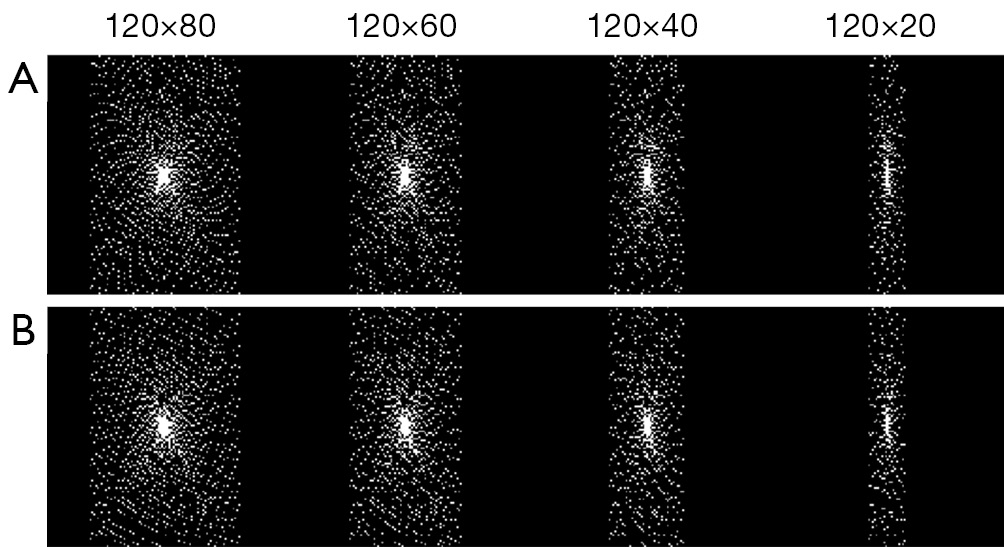Figure 5 Representative undersampling patterns of asymmetric sizes 128×80, 128×60, 128×40 and 128×20 with (A) Eq.  (b=30) and (B) Eq.  (c=1.5) respectively.

### Retrospective undersampling

In this study, fully sampled data sets were acquired and used for evaluating our proposed CIRCUS patterns and other often used undersampling patterns. The data sets included numerical and experimental phantoms, as well as human images. A Shepp-Logan numerical phantom (MATLAB) was simulated to generate a 200×200 image and its k-space data on ky-kz plane. Water structure phantoms were scanned with a 3D gradient-echo sequence on a GE 3.0 T scanner (GE Medical Systems, Milwaukee, WI) with the body coil and with a 12-channel head coil respectively. In both scan time was around one minute, and the image matrices were 128×128×128, with an isotropic resolution of 1.5 mm. Healthy volunteers were scanned on a Siemens 3.0 T scanner (Siemens Medical Systems, Erlangen, Germany). Neck image data was acquired using a 3D Fast Low Angle Shot (FLASH) sequence and a 20-channel coil. Scan time was around 1.7 minutes. Fully sampled data had an image matrix of 340×256×128 and every other line along ky direction was removed to have a symmetric ky-kz encoding matrix 128×128. Brain image data was acquired with a 3D Magnetization Prepared Rapid Acquisition Gradient Echo (MP-RAGE) sequence and a 16-channel coil. Scan time was around 7.5 minutes. Fully sampled data was originally 512×256×176, and truncated to have a symmetric ky-kz encoding matrix of 176×176.

### Sampling schemes and image reconstruction

We compared the CIRCUS patterns with three currently used random sampling patterns, including the conventional uRS, advanced PDS and vPDS. All the algorithms for generating sampling patterns were implemented using Matlab (The MathWorks, Natick, MA). For uRS, PDS and vPDS patterns, a Matlab function that generates uniformly distributed pseudorandom numbers was applied. To account for random variation, the algorithms for generating uRS, PDS and vPDS patterns were each repeated ten times independently. We applied CIRCUS to generate various sampling patterns with golden-ratio profiles, including CIRCUS radial and spiral patterns. CIRCUS radial patterns were generated with Eq. , with ten different values of b (0, 1, 10 to 80 with an incensement of 10). CIRCUS spiral patterns were generated with Eq. , with ten different values of c (1.0 to 1.9 with an incensement of 0.1). The tendency of each group of the sampling patterns is to have an increasing degree of sampling randomization. The five categories of sampling methods described above (uRs, PDS, vPDS, CIRCUS radial, and CIRCUS spiral) have been applied to achieve the same acceleration factor of R=6. For three selected variable-density sampling schemes vPDS, CIRCUS radial (b=40), and CIRCUS spiral (c=1.5), we applied a series of different acceleration factors R=2 to 8 for further evaluation and comparisons. For all the sampling schemes described above, a fully sampled k-space center, covering a square of size ceil (0.12× N), was acquired for coil calibration in PI reconstruction. The high-frequency points outside a disc region of diameter N were skipped.

All sampling patterns were retrospectively applied to the fully sampled data sets. CS image reconstruction (4) was applied for single coil data sets, including numerical and experimental phantoms, as well as multi-channel brain images; SPIRiT image reconstruction (7) was applied for data sets with multi-channels, including experimental phantom and human imaging.

### Data analysis

Normalized root-mean-square errors (NRMSE) were measured from images obtained with the undersampling patterns compared to those obtained with the fully sampled data sets (termed as the reference images): , where Iref and Iacc are the images obtained with full sampled data sets and accelerated sampling data sets respectively, and is the Euclidean norm.

### Prospective implementation

The proposed CIRCUS sampling strategy (both radial and spiral types) was implemented in a 3D gradient-echo sequence. To evaluate eddy-current related effects on the balanced Steady-State Free Procession (bSSFP) sequence caused by the proposed sampling pattern and order (26), data was acquired from a spherical phantom filled with doped water on a 3.0 T MR scanner (GE Medical Systems, Milwaukee, WI) with an 8-channel cardiac coil. The scan parameters were: FOV =320 mm, TR/TE =4.3/1.2 ms, FA =45°, BW =±125 kHz, slice thickness of 5 mm, image matrix =256×160×40. The acceleration factor was R=4, and CS was applied for image reconstruction. Data from the conventional full sampling was also acquired as reference. Brain images were acquired with bSSFP, FOV =192 mm, TR/TE =4.4/1.7 ms, FA =45°, BW =±125 kHz, slice thickness of 2.5 mm, image matrix =160×160×64 (partial ky and kz acquisition, and corner cutting). The acceleration factor was R=4 and CS was applied for image reconstruction. Reference images were also obtained.

## Results

Figure 6 shows the NRMSEs measured from images obtained with the undersampling patterns versus the reference images. Three cases were reconstructed with CS (Figure 6A-C) and three cases were reconstructed with SPIRiT (Figure 6D-F), all with an acceleration factor of R=6. The horizontal bars with uRS, PDS and vPDS patterns correspond to the averaged errors over ten independently repeated sampling patterns. As demonstrated by others, CS with variable-density random sampling generally outperforms that with uRS (4). As shown in Figure 6A-C, for CS image reconstruction, the image reconstruction errors with uRS (red dots) and PDS (green dots) are generally higher than those for the other variable-density sampling methods, and the degree of sampling randomization affects the image reconstruction accuracy but does not contribute significantly. For the SPIRiT image reconstruction, the randomization of the sampling points plays an important role as shown in Figure 6D-F, where the undersampling patterns with higher degree of randomization provide higher accuracy of image reconstruction.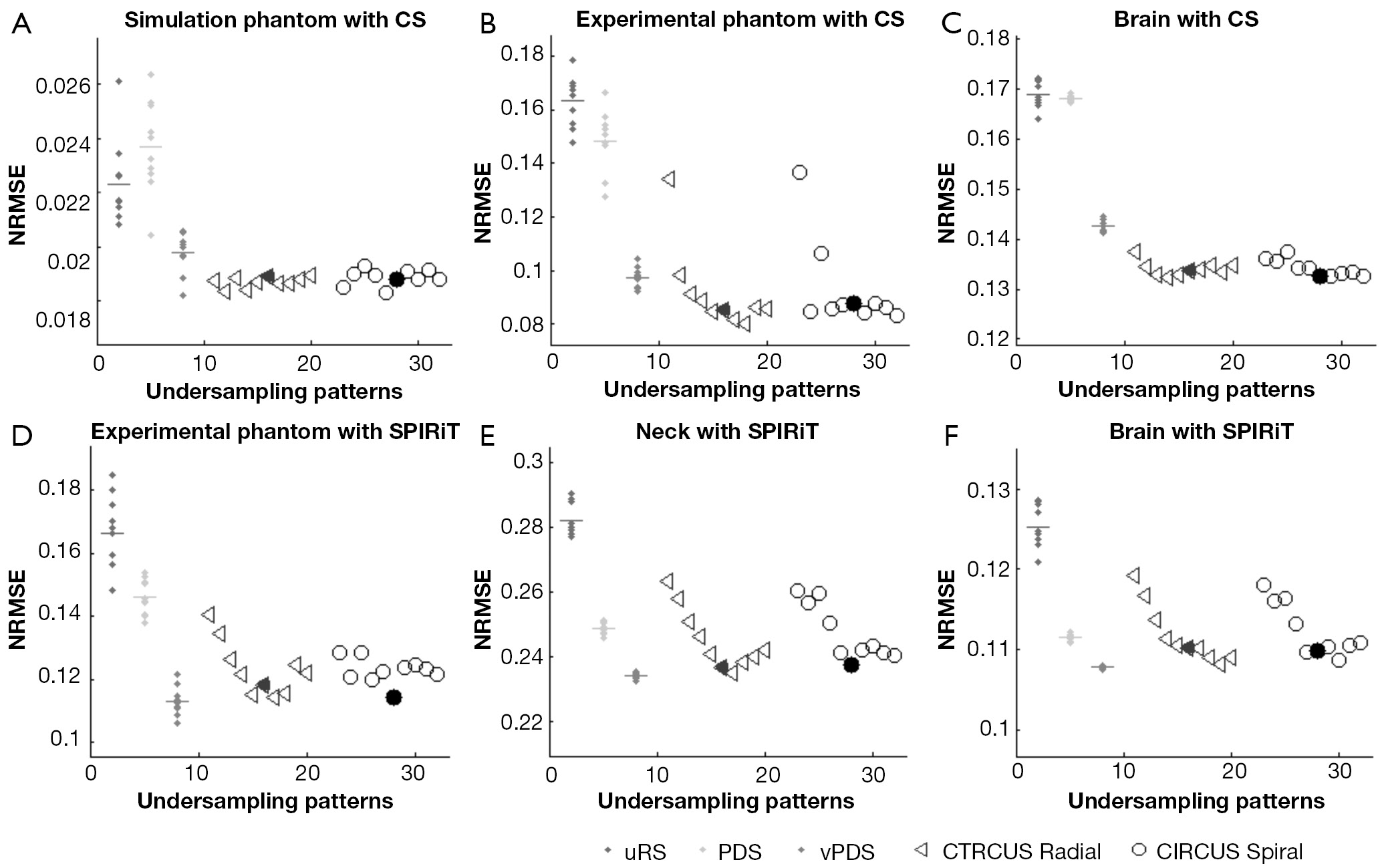Figure 6 Image reconstruction errors for different sampling patterns: uRS (red dots, ten repeats), PDS (green dots, ten repeats), vPDS (magenta dots, ten repeats), CIRCUS radial {blue triangles, Eq.  with b=0, 1, 10 to 80 with an incensement of 10} and spiral {black circles, Eq.  with c=1.0 to 1.9 with an incensement of 0.1}, for three subjects with CS (A-C) and three subjects with SPIRiT (D-F). Averaged errors for uRS, PDS and vPDS were plotted as short horizontal lines. Solid triangle {Eq.  with b=40} and circle markers {Eq.  with c=1.5} are highlighted as the selected CIRCUS patterns that provide sufficient randomization and reliable image reconstruction.

Based on the CIRCUS sampling patterns (with different degrees of randomization) (Figures 2,3) and performance of the image reconstruction (Figure 6), certain values of the parameters b and c in Eqs. [2,3 could be found to generate reliable CIRCUS sampling patterns for the CS and SPIRiT methods. In Figure 6, the NRMSEs with the selected values, b=40 {CIRCUS radial, Eq. } and c=1.5 {CIRCUS spiral, Eq. }, are indicated with solid markers, and consistently provide high accuracy of image reconstruction, regardless of imaging subjects or reconstruction methods. Figure 7 shows the representative sampling patterns with uRS (Figure 7A), PDS (Figure 7B) and vPDS (Figure 7C), and the selected sampling patterns with CIRCUS radial {Eq. , b=40} (Figure 7D) and CIRCUS spiral {Eq. , c=1.5} (Figure 7E), all with the same acceleration factor of R=6. The reference image and images reconstructed with the corresponding undersampling patterns are plotted in Figure 8 with CS (A, numerical phantom; B, experimental phantom; and C, brain), and in Figure 9 with SPIRiT (A, experimental phantom; B, neck; and C, brain). This demonstrates that randomized CIRCUS patterns provide reliable performance, similar (with SPIRiT) or perhaps even better (with CS) than those with vPDS.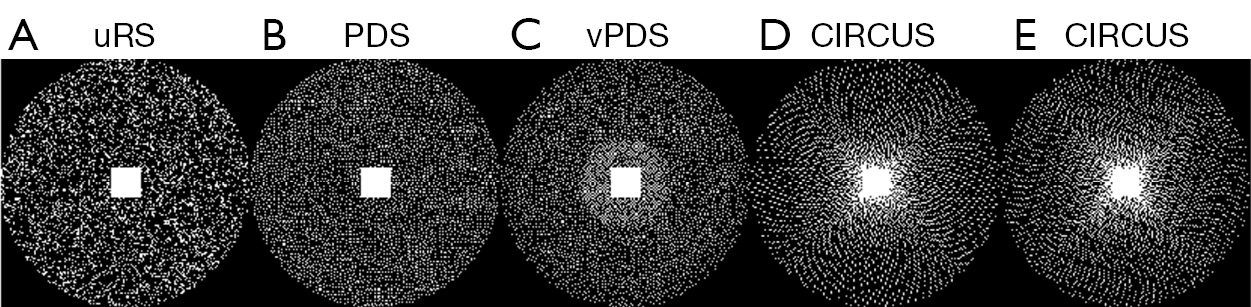Figure 7 Undersampling patterns with (A) uRS, (B) PDS, (C) vPDS, (D) CIRCUS radial {Eq.  with b=40} and (E) CIRCUS spiral {Eq.  with c=1.5}. All achieve the same acceleration factor of R=6 given a 200×200 ky-kz sampling matrix.Figure 8 Comparisons with CS reconstruction. (A) Numerical phantom; (B) experimental phantom; and (C) brain images were reconstructed with CS using fully sampled data sets, undersampled data sets (R=6) with sampling patterns uRS, PDS, vPDS, CIRCUS radial {Eq.  with b=40} and spiral {Eq.  with c=1.5}. The second row of each block shows the difference images between the images with acceleration and the reference image, with a rescaled window level. The NRMSEs are displayed at the bottom-left corner of the difference images.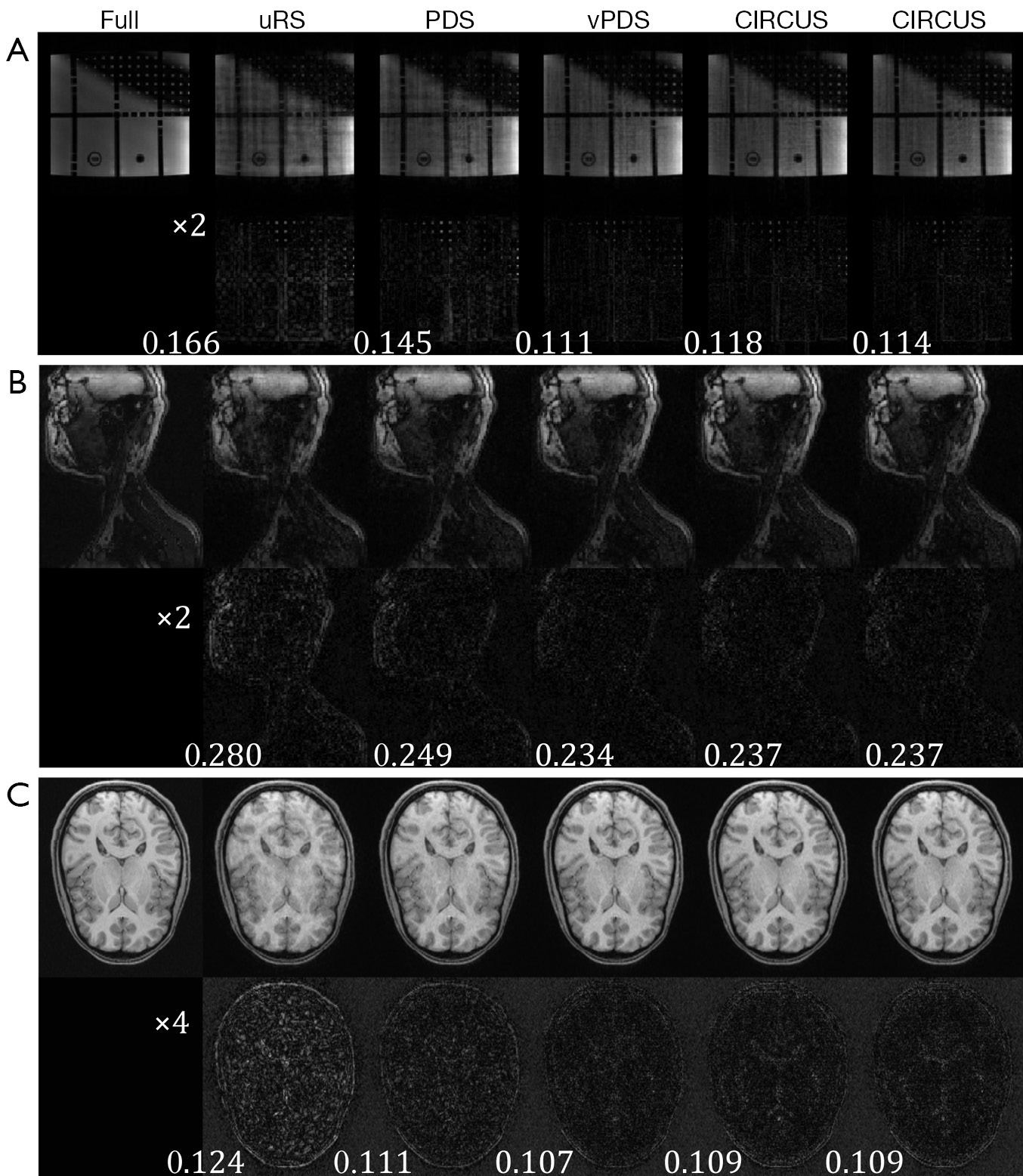Figure 9 Comparisons with PI reconstruction. (A) Experimental phantom; (B) neck; and (C) brain images reconstructed with SPIRiT using fully sampled data sets, undersampled data sets (R=6) with sampling patterns uRS, PDS, vPDS, CIRCUS radial {Eq.  with b=40} and spiral {Eq.  with c=1.5}. The second row of each block shows the difference images between the images with acceleration and the reference image, with a rescaled window level. The NRMSEs are displayed at the bottom-left corner of the difference images.

We compared the image reconstruction accuracy obtained with vPDS, the selected randomized CIRCUS patterns including CIRCUS radial {Eq. , b=40} and CIRCUS spiral {Eq. , c=1.5}, for all the six cases (as in Figure 6) and with different undersampling factors ranging from 4 to 8. Figure 10 plots the NRMSEs of the three promising sampling schemes, demonstrating that our proposed randomized CIRCUS methods provide consistently comparable results to vPDS for different undersampling factors.Figure 10 Image reconstruction errors with different sampling patterns: vPDS (red dots, ten repeats), CIRCUS radial {blue triangles, Eq.  with b=40} and spiral {black circles, Eq.  with c=1.5}, at different acceleration factors (4 to 8), for three subjects with CS (A-C) and three subjects with SPIRiT (D-F). Short red horizontal lines indicate the averaged errors for vPDS.

Figure 11 shows the reference image and those obtained with prospectively implemented CIRUCS (R=4) in a 3D bSSFP sequence. Compared to CIRCUS radial, CIRCUS spiral pattern has a smoother transition for the sampling order. Thus CIRCUS spiral provides comparable image quality to that of the reference image, while CIRCUS radial may suffer obvious eddy-current related artifacts, as demonstrated in the spherical phantom (Figure 11A).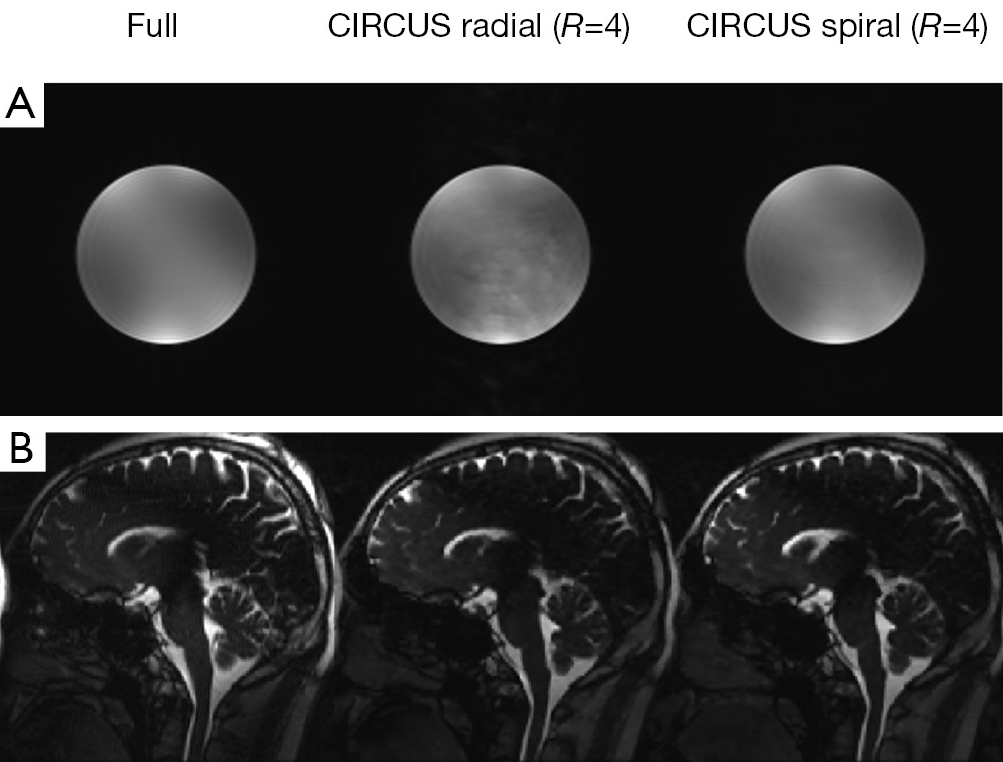Figure 11 Phantom and brain images with full, CIRCUS radial (b=40, R=4), and CIRCUS spiral (c=1.5, R=4) sampling.

## Discussion and conclusions

It has been shown that CIRCUS can generate various sampling patterns including radial, spiral or randomized patterns on the ky-kz plane of 3D Cartesian acquisitions. It easily controls the degree of undersampling and randomization, but maintains desirable features of the radial or spiral sampling such as interleaving flexibility. We investigated the parameters that adjust the randomization and radial or spiral features, and identified specific values that provide reliable image reconstructions. Our results show that the selected randomized CIRCUS radial and spiral patterns are sufficiently randomized similar to vPDS, while retaining sampling flexibility similar to that with radial or spiral sampling. The image reconstructions outperform the uniform random sampling, are comparable to the vPDS, but maintain good sampling flexibility. Similar to the conventional non-Cartesian radial or spiral acquisition, CIRCUS repeats acquisition of low frequency data, thus the undersampling efficiency is reduced accordingly. For a reasonable acceleration factor (4 to 8), the efficiency reduction is less than 10%.

For images acquired with bSSFP sequence, specific sampling orders may enhance or compensate eddy-current related artifacts (26). Since the CIRCUS spiral pattern has a smooth transition of the sampling trajectories, it may be less sensitive to the eddy-current effects compared to CIRCUS radial. Obvious artifacts appeared in the spherical phantom image obtained with CIRCUS radial (Figure 11A), although those were less visible in the brain image (Figure 11B).

In conclusion, this study proposed a novel method, CIRcular Cartesian UnderSampling (CIRCUS), for generating undersampling patterns for 3D Cartesian imaging using CS or PI reconstruction methods. Reliable image reconstructions with the proposed randomized CIRCUS patterns have been demonstrated to be better than those with uniform random samplings, and were found to be comparable to those obtained with vPDS. Further evaluations of using CIRCUS strategy for specific applications, especially dynamic imaging, will be investigated.

## Acknowledgements

This work was supported in part by grants from the NIH K25EB014914 (Jing Liu), R01NS059944 (David Saloner), and a VA MERIT review grant (David Saloner).

Disclosure: The authors declare no conflict of interest.

1. Donoho DL. Compressed sensing. IEEE Trans Inf Theory 2006;52:1289-306.
2. Candes EJ, Romberg J, Tao T. Robust uncertainty principles: exact signal reconstruction from highly incomplete frequency information. IEEE Trans Inf Theory 2006;52:489-509.
3. Candes EJ, Tao T. Near-optimal signal recovery from random projections: Universal encoding strategies? IEEE Trans Inf Theory 2006;52:5406-25.
4. Lustig M, Donoho D, Pauly JM. Sparse MRI: the application of compressed sensing for rapid MR imaging. Magn Reson Med 2007;58:1182-95. [PubMed]
5. Pruessmann KP, Weiger M, Scheidegger MB, et al. SENSE: sensitivity encoding for fast MRI. Magn Reson Med 1999;42:952-62. [PubMed]
6. Griswold MA, Jakob PM, Heidemann RM, et al. Generalized autocalibrating partially parallel acquisitions (GRAPPA). Magn Reson Med 2002;47:1202-10. [PubMed]
7. Lustig M, Pauly JM. SPIRiT: Iterative self-consistent parallel imaging reconstruction from arbitrary k-space. Magn Reson Med 2010;64:457-71. [PubMed]
8. Cook RL. Stochastic sampling in computer-graphics. ACM Trans Graph 1986;5:51-72.
9. Dunbar D, Humphreys G. A spatial data structure for fast Poisson-disk sample generation. ACM Trans Graph 2006;25:503-8.
10. Vasanawala S, Murphy M, Alley M, et al. Practical parallel imaging compressed sensing MRI: Summary of two years of experience in accelerating body MRI of pediatric patients. Proc IEEE Int Symp Biomed Imaging 2011;2011:1039-43.
11. Bridson R. Fast Poisson disk sampling in arbitrary dimensions. ACM SIGGRAPH 2007 Sketches.
12. Glover GH, Pauly JM. Projection reconstruction techniques for reduction of motion effects in MRI. Magn Reson Med 1992;28:275-89. [PubMed]
13. Peters DC, Korosec FR, Grist TM, et al. Undersampled projection reconstruction applied to MR angiography. Magn Reson Med 2000;43:91-101. [PubMed]
14. Ahn CB, Kim JH, Cho ZH. High-speed spiral-scan echo planar NMR imaging-I. IEEE Trans Med Imaging 1986;5:2-7. [PubMed]
15. Liao JR, Pauly JM, Brosnan TJ, et al. Reduction of motion artifacts in cine MRI using variable-density spiral trajectories. Magn Reson Med 1997;37:569-75. [PubMed]
16. Börnert P, Stuber M, Botnar RM, et al. Direct comparison of 3D spiral vs. Cartesian gradient-echo coronary magnetic resonance angiography. Magn Reson Med 2001;46:789-94. [PubMed]
17. Haider CR, Hu HH, Campeau NG, et al. 3D high temporal and spatial resolution contrast-enhanced MR angiography of the whole brain. Magn Reson Med 2008;60:749-60. [PubMed]
18. Du J. Contrast-enhanced MR angiography using time resolved interleaved projection sampling with three-dimensional Cartesian phase and slice encoding (TRIPPS). Magn Reson Med 2009;61:918-24. [PubMed]
19. Doneva M, Stehning C, Nehrke K, et al. Improving scan efficiency of respiratory gated imaging using compressed sensing with 3D Cartesian golden angle sampling. Proc Intl Soc Mag Reson Med 2011;19:641.
20. Winkelmann S, Schaeffter T, Koehler T, et al. An optimal radial profile order based on the Golden Ratio for time-resolved MRI. IEEE Trans Med Imaging 2007;26:68-76. [PubMed]
21. Kim YC, Narayanan SS, Nayak KS. Flexible retrospective selection of temporal resolution in real-time speech MRI using a golden-ratio spiral view order. Magn Reson Med 2011;65:1365-71. [PubMed]
22. Lin W, Guo J, Rosen MA, et al. Respiratory motion-compensated radial dynamic contrast-enhanced (DCE)-MRI of chest and abdominal lesions. Magn Reson Med 2008;60:1135-46. [PubMed]
23. Liu J, Spincemaille P, Codella NC, et al. Respiratory and cardiac self-gated free-breathing cardiac CINE imaging with multiecho 3D hybrid radial SSFP acquisition. Magn Reson Med 2010;63:1230-7. [PubMed]
24. Uribe S, Muthurangu V, Boubertakh R, et al. Whole-heart cine MRI using real-time respiratory self-gating. Magn Reson Med 2007;57:606-13. [PubMed]
25. Noll DC, Nishimura DG, Macovski A. Homodyne detection in magnetic resonance imaging. IEEE Trans Med Imaging 1991;10:154-63. [PubMed]
26. Bieri O, Markl M, Scheffler K. Analysis and compensation of eddy currents in balanced SSFP. Magn Reson Med 2005;54:129-37. [PubMed]
Cite this article as: Liu J, Saloner D. Accelerated MRI with CIRcular Cartesian UnderSampling (CIRCUS): a variable density Cartesian sampling strategy for compressed sensing and parallel imaging. Quant Imaging Med Surg 2014;4(1):57-67. doi: 10.3978/j.issn.2223-4292.2014.02.01